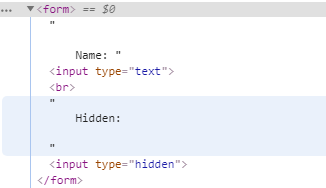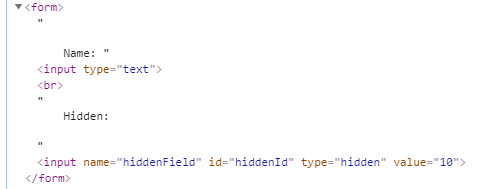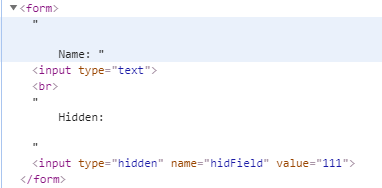# How to create hidden form element on the fly using jQuery ?

• Difficulty Level : Easy
• Last Updated : 31 Oct, 2019

JQuery is the library that makes the use of JavaScript easy. Inserting the <input type=’hidden’> can also be done using jQuery. The append() and appendTo() inbuilt function can be used to add the hidden form element but they are not only limited to the <input type=’hidden’> but we can also add other html elements.

Note: They both perform pretty much the same. The major difference is only about the syntax and that is explained below.

Using appendTo() method: In the appendTo() method, the content comes before the method like `\$(content).appendTo(selector)`.

Below examples illustrates the appendTo() method to create hidden form element:

Example 1: If only one attribute is to be added, then it can be given by passing two arguments in attr() method. First argument is the name of attribute, and the second argument is the value of the attribute.

 ``` ` `<``html``>`` ` `<``head``>``    ``<``title``>GeeksforGeeks `` ` `    ``<``script` `src``=``"https://code.jquery.com/jquery-3.4.1.js"` `integrity``=``"sha256-WpOohJOqMqqyKL9FccASB9O0KwACQJpFTUBLTYOVvVU="` `            ``crossorigin``=``"anonymous"``>``    `````` ` `<``body``>``    ``<``script``>``        ``\$(document).ready(function() {``            ``\$("<``input``>").attr("type", "hidden").appendTo("form");``        ``})``    ```` ` `    ``<``form``>`` ` `        ``Name:``        ``<``input` `type``=``"text"``>``        ``<``br``> Hidden:`` ` `    ```` ` ``` ` ``

Output: The output can be seen in the browser using Inspect Element feature (e.g. ctrl + shift + i in Google Chrome).Example 2: Multiple attributes can also be given by passing them as an object of attributes in the attr() method.

 ```<``html``>`` ` `<``head``>``    ``<``title``>GeeksforGeeks`` ` `    ``<``script` `src``=``"https://code.jquery.com/jquery-3.4.1.js"` `integrity``=``"sha256-WpOohJOqMqqyKL9FccASB9O0KwACQJpFTUBLTYOVvVU="` `            ``crossorigin``=``"anonymous"``>``    ```` ` ``` ` `<``body``>``    ``<``script``>``        ``\$(document).ready(function() {``            ``\$("<``input``>").attr({``                ``name: "hiddenField",``                ``id: "hiddenId",``                ``type: "hidden",``                ``value: 10``            ``}).appendTo("form");`` ` `        ``})``    ```` ` `    ``<``form``>`` ` `        ``Name:``        ``<``input` `type``=``"text"``>``        ``<``br``> Hidden:`` ` `    ```` ` ``` ` ``

Output: The output can be seen in the browser using Inspect Element feature (e.g. ctrl + shift + i in Google Chrome).Using append() method: In the append() method, the content comes after the method like `\$(selector).append(content) `.

Below example illustrates the append() method to create hidden form element:

Example:

 ``` ` `<``html``>`` ` `<``head``>``    ``<``title``>GeeksforGeeks`` ` `    ``<``script` `src``=``"https://code.jquery.com/jquery-3.4.1.js"` `integrity``=``"sha256-WpOohJOqMqqyKL9FccASB9O0KwACQJpFTUBLTYOVvVU="` `            ``crossorigin``=``"anonymous"``>`` ` ``` ` `<``body``>``    ``<``script``>``        ``\$(document).ready(function() {``            ``\$("form").append("<``input` `type``=``'hidden'``                    ``name``=``'hidField'` `value``=``'111'``>")``        ``})``    ```` ` `    ``<``form``>`` ` `        ``Name:``        ``<``input` `type``=``"text"``>``        ``<``br``> Hidden:`` ` `    ```` ` ``` ` ``

Output: The output can be seen in the browser using Inspect Element feature (e.g. `ctrl + shift + i` in Google Chrome).My Personal Notes arrow_drop_up